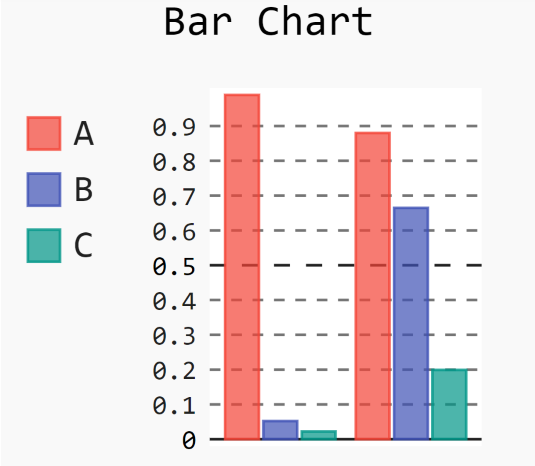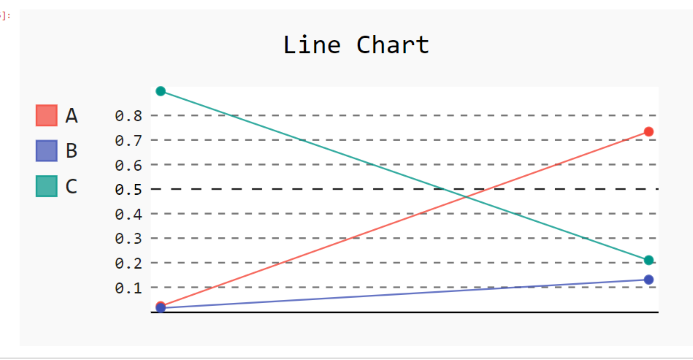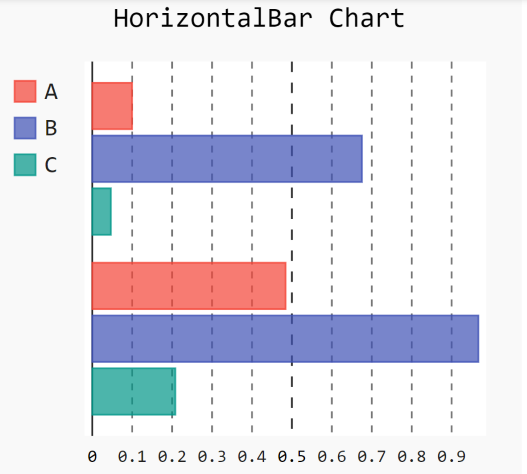# How to limit the width and height in Pygal?

• Last Updated : 24 Jan, 2021

Prerequisites: pygal

Pygal is a graphics and user interface library for Python that provides functionality commonly required in designing and science applications. While making a plot it is important for us to optimize its size. In this article, we will see how we can resize the plot window in the Pygal module. Here are various ways to change the default plot size as per our required dimensions or resize a given plot.

Attention geek! Strengthen your foundations with the Python Programming Foundation Course and learn the basics.

To begin with, your interview preparations Enhance your Data Structures concepts with the Python DS Course. And to begin with your Machine Learning Journey, join the Machine Learning - Basic Level Course

Approach:

• Import required module.
• Create a chart object.
• Pass width/height in function.
• Label the graph.
• Display Graph.

Implementation of the concept discussed above is given below:

Example 1:

## Python3

 `# importing pygal``import` `pygal``import` `numpy`` ` `# creating the chart object``chart ``=` `pygal.Bar(width``=``200``, height``=``200``)`` ` `# naming the title``chart.title ``=` `'Bar Chart'`` ` `# Random data``chart.add(``'A'``, numpy.random.rand(``2``))``chart.add(``'B'``, numpy.random.rand(``2``))``chart.add(``'C'``, numpy.random.rand(``2``))`` ` `chart.render_to_png(``'img.png'``)`

Output:Example 2:

## Python3

 `# importing pygal``import` `pygal``import` `numpy`` ` `# creating the chart object``chart ``=` `pygal.Line(width``=``400``, height``=``200``)`` ` `# naming the title``chart.title ``=` `'Line Chart'`` ` `# Random data``chart.add(``'A'``, numpy.random.rand(``2``))``chart.add(``'B'``, numpy.random.rand(``2``))``chart.add(``'C'``, numpy.random.rand(``2``))`` ` `chart.render_to_png(``'img.png'``)`

Output:Example 3:

## Python3

 `# importing pygal``import` `pygal``import` `numpy`` ` `# creating the chart object``chart ``=` `pygal.HorizontalBar(width``=``300``, height``=``300``)`` ` `# naming the title``chart.title ``=` `'HorizontalBar Chart'`` ` `# Random data``chart.add(``'A'``, numpy.random.rand(``2``))``chart.add(``'B'``, numpy.random.rand(``2``))``chart.add(``'C'``, numpy.random.rand(``2``))`` ` `chart.render_to_png(``'img.png'``)`

Output:My Personal Notes arrow_drop_up